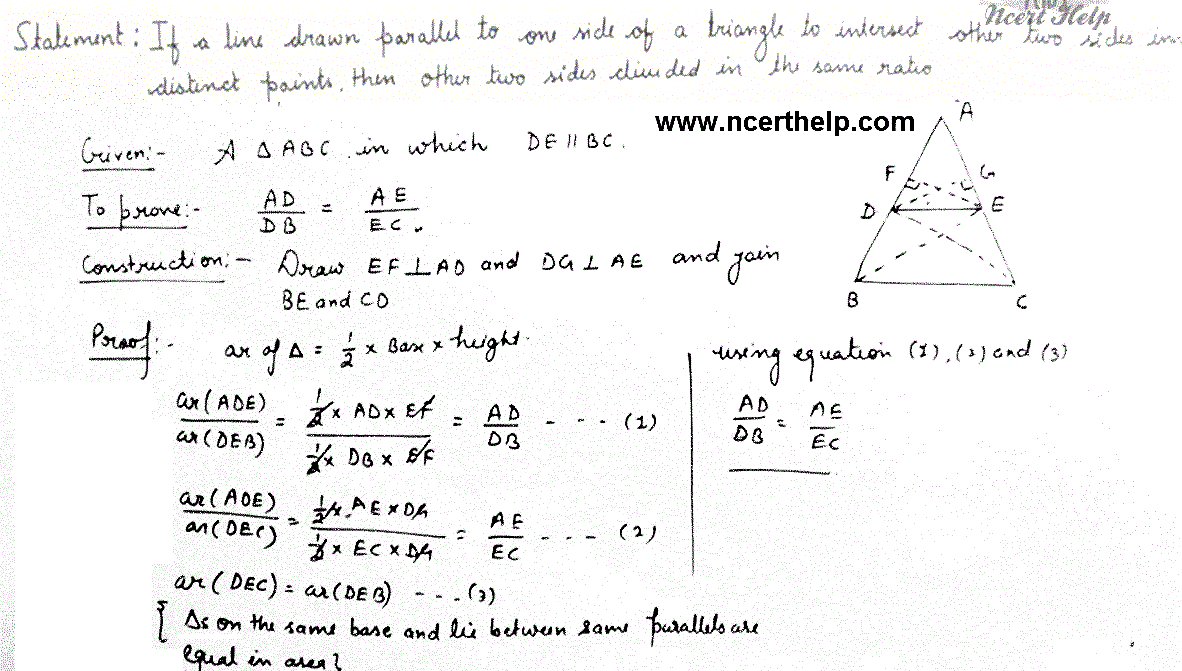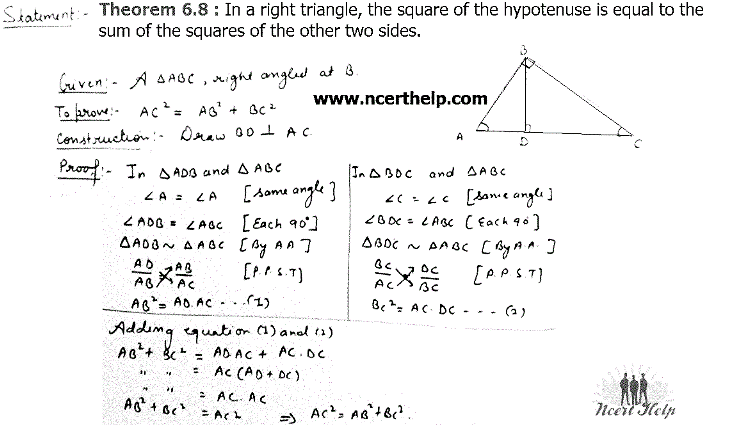# NCERT Solutions Class 10 Maths Chapter 6 Triangles

Ncert Solutions for class 10 subject Maths Chapter 6 Trianglesin pdf Best Free NCERT Solutions for class 1 to 12 in pdf NCERT Solutions, cbse board, Maths, ncert Solutions for Class 10 Maths, class 10 Maths ncert solutions, Triangles, Class 10, ncert solutions chapter 6 Triangles, class 10 Maths, class 10 Maths ncert solutions, Maths ncert solutions class 10, Ncert Solutions Class 10 Mathematics Chapter 6 Triangles

## Theorems Chapter 6 Class 10 Maths Triangles Ncert Solutions

Theorem 6.1: If a line is drawn parallel to one side of a triangle to intersect the other two sides in distinct points, the other two sides are ided in the same ratio.Theorem 6.8 : In a right triangle, the square of the hypotenuse is equal to the sum of the squares of the other two sides.### NCERT Books Free Pdf Download for Class 5, 6, 7, 8, 9, 10 , 11, 12 Hindi and English Medium

 Mathematics Biology Psychology Chemistry English Economics Sociology Hindi Business Studies Geography Science Political Science Statistics Physics Accountancy